﻿ 船舶重心位置对固有频率影响规律研究
 舰船科学技术2023, Vol. 45Issue (1): 14-17    DOI: 10.3404/j.issn.1672-7649.2023.01.003PDF

, 叶仁传, 陈运, 刘虎

Study on the effect of barycenter position of ship on natural frequency
ZHANG Zhi-hao, YE Ren-chuan, CHEN Yun, LIU Hu
School of Naval Architecture and Ocean Engineering, Jiangsu University of Science and Technology, Zhenjiang 212003, China
Abstract: The adjustment of the center of gravity in the finite element analysis of the ship is time-consuming and laboring, so this paper studies the influence degree of the whole ship's center of gravity on the natural frequency results based on the finite element method, by comparing the variation trend of natural frequency at different height of center of gravity, the correctness of the results was verified by finite element method and empirical formula method. Through the comparison of the cylindrical example, the basic law is obtained, and then the natural frequency of the whole ship is calculated, and the following conclusions are drawn: In a certain range, the natural frequency will decrease with the increase of the height of the center of gravity, and tends to be flat after a sharp change. Therefore, the relation between the result of the center of gravity of the whole ship and the natural frequency can be used to guide the adjustment range of the center of gravity of the whole ship in the calculation according to the finite element.
Key words: added mass     finite element method     natural frequency     effect of center of gravity
0 引　言

1 理论与数值计算 1.1 经验公式估算

 ${N_{V2}} = C\sqrt {{I_V}/{\Delta _V}{L^3}} \times 0.0167。$ (1)

 \begin{aligned}[b] {N_{V2}} =& 3409920 \sqrt {9.7825/6422\times{{112.7}^3}} \times 0.0167 = \\ & 1.8577\;{\rm{Hz}}。\end{aligned} (2)

 ${N_{V3}} = {(3 - 1)^{1.02}}{N_{V2}} = 3.7673\; {\rm{Hz}}。$ (3)

 ${f_{ih}} = {a_{ih}}{K_{ih}}{E_{ih}}\sqrt {{I_{oh}}/{\Delta _h}{L^3}} + {b_{ih}}(i=2)，$ (4)
 $\begin{split} {f_{2h}} = &0.473 \times {10^5} \times 0.943 \times 1 \times \sqrt {31.8111/6422 \times {{112.7}^3}} +\\ & 0.362 = 2.9859\;{\rm{Hz}}，\\[-10pt] \end{split}$ (5)
 ${f}_{3h}=(3-1{)}^{1.02}\times 2.9859=6.0552 \;{\rm{Hz}}。$ (6)
1.2 附加质量法

 $T = \frac{1}{2}\rho \text{π} {b^2}{v^2}。$ (7)

$\rho \text{π} {b^2} = M$ $M$ 为单位长度的液体质量，可得：

 $T = \frac{1}{2}M{v^2} 。$ (8)

 $M = \text{π} c\rho {b^2} 。$ (9)

 ${M_\alpha }V = \frac{1}{2}\text{π} {\alpha _V}{C_V}{K_i}\rho {B^2} 。$ (10)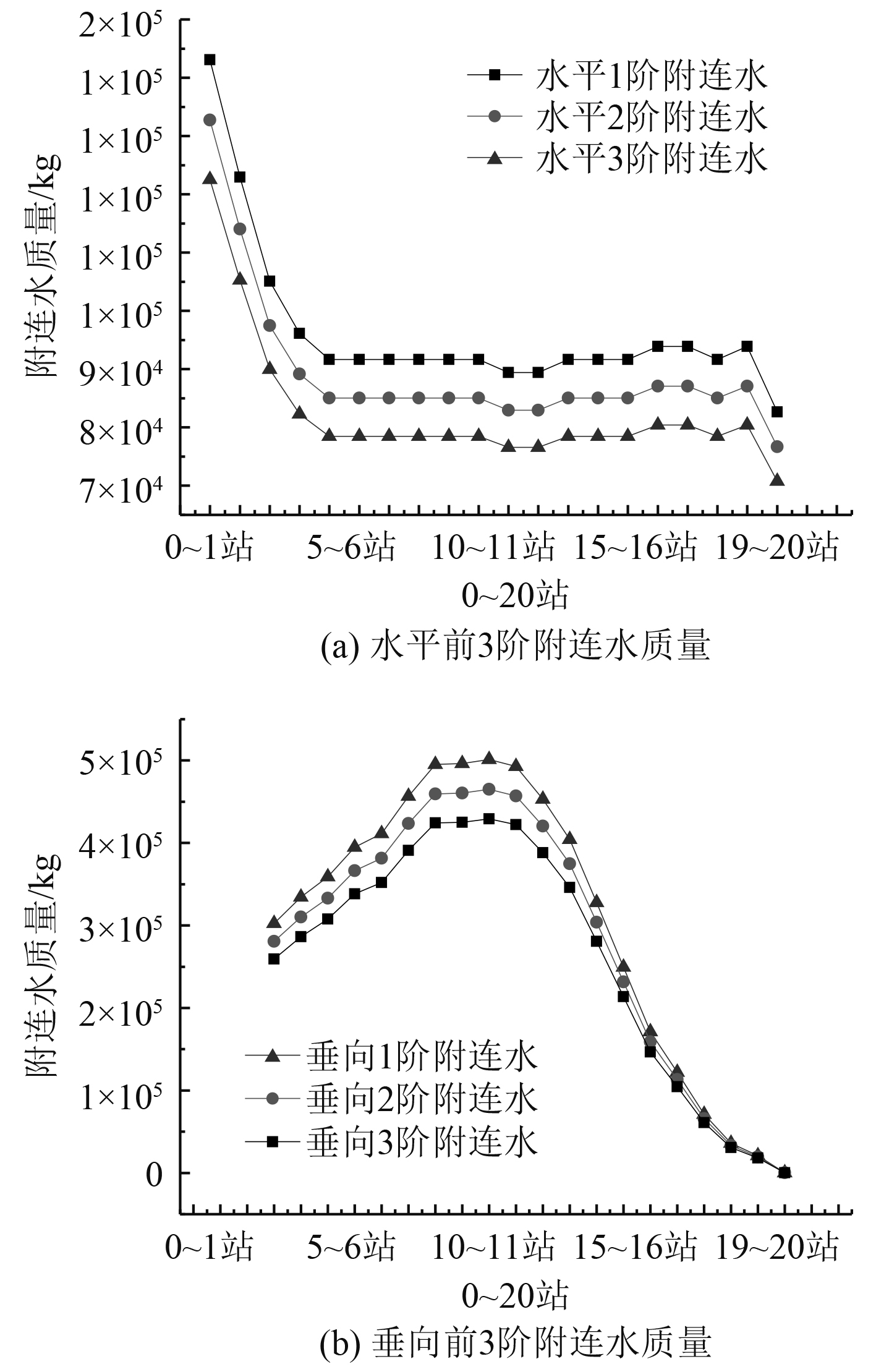图 1 前3阶附连水质量 Fig. 1 The first three attached water masses
1.3 声固耦合法

2 有限元计算分析 2.1 有限元算例验证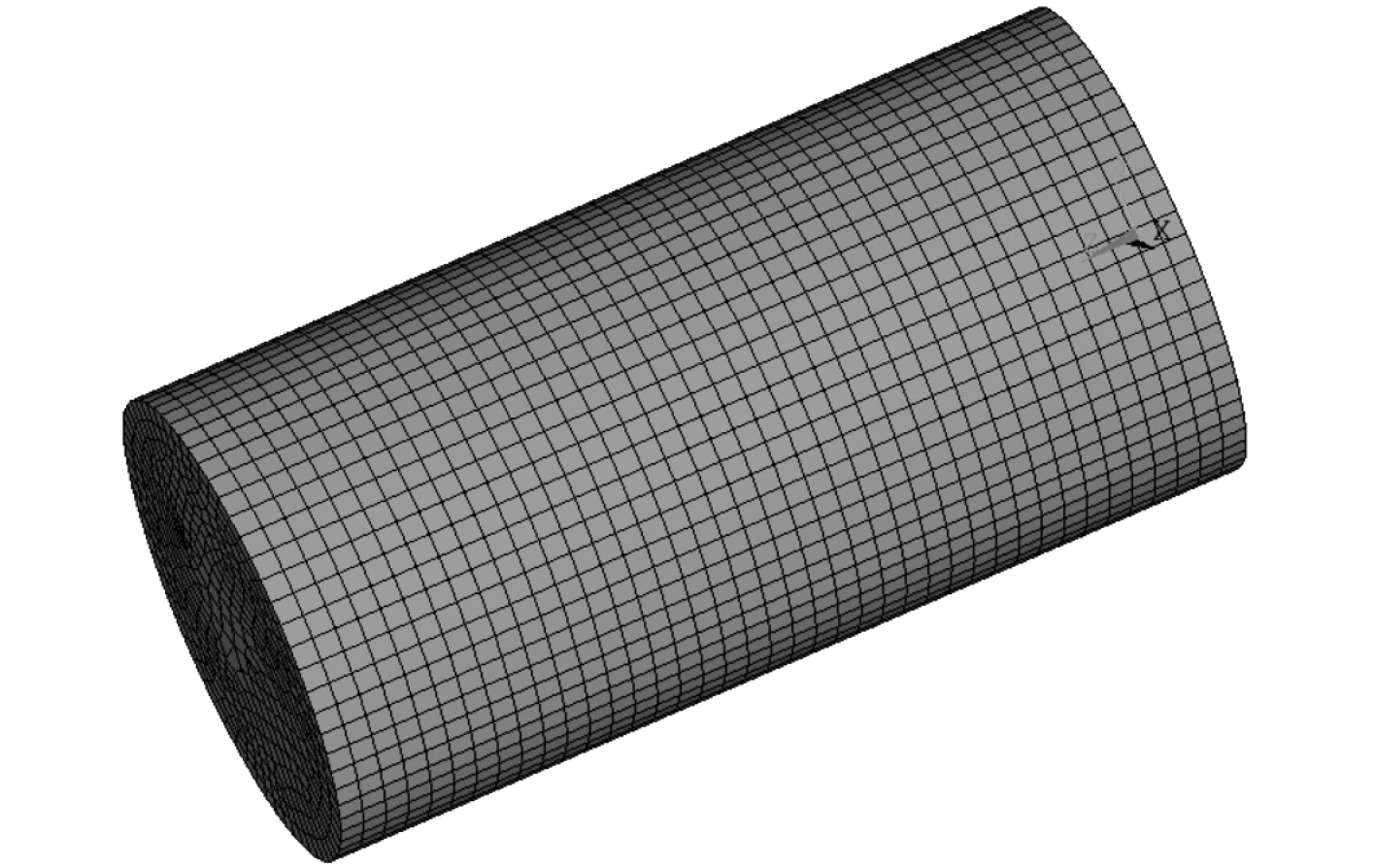图 2 圆柱算例有限元模型 Fig. 2 Finite element model of cylindrical example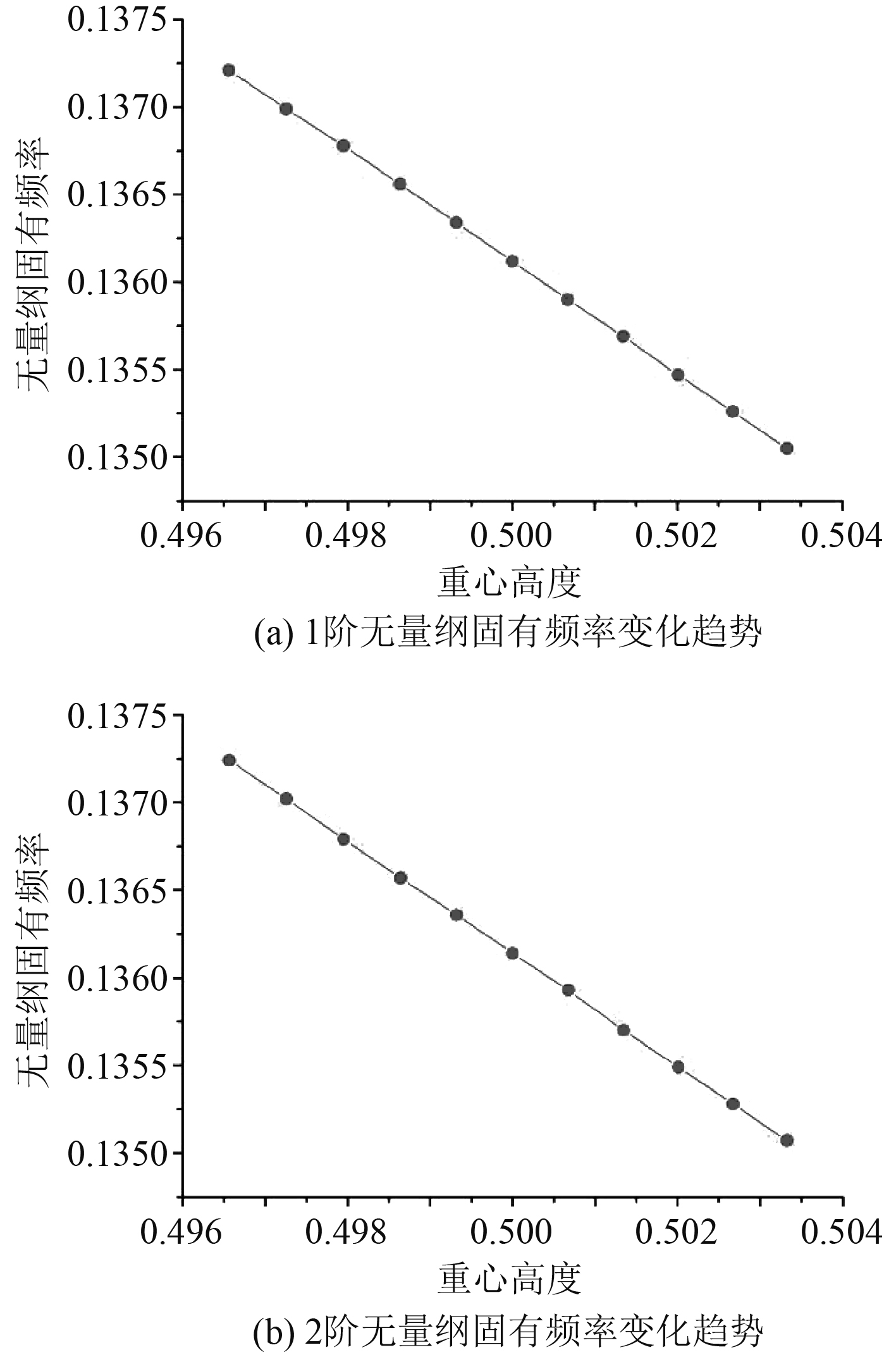图 3 实心圆柱前2阶固有频率随重心高度的变化曲线 Fig. 3 The natural frequencies of the first two orders of the solid cylinder vary with the height of the center of gravity
2.2 有限元模型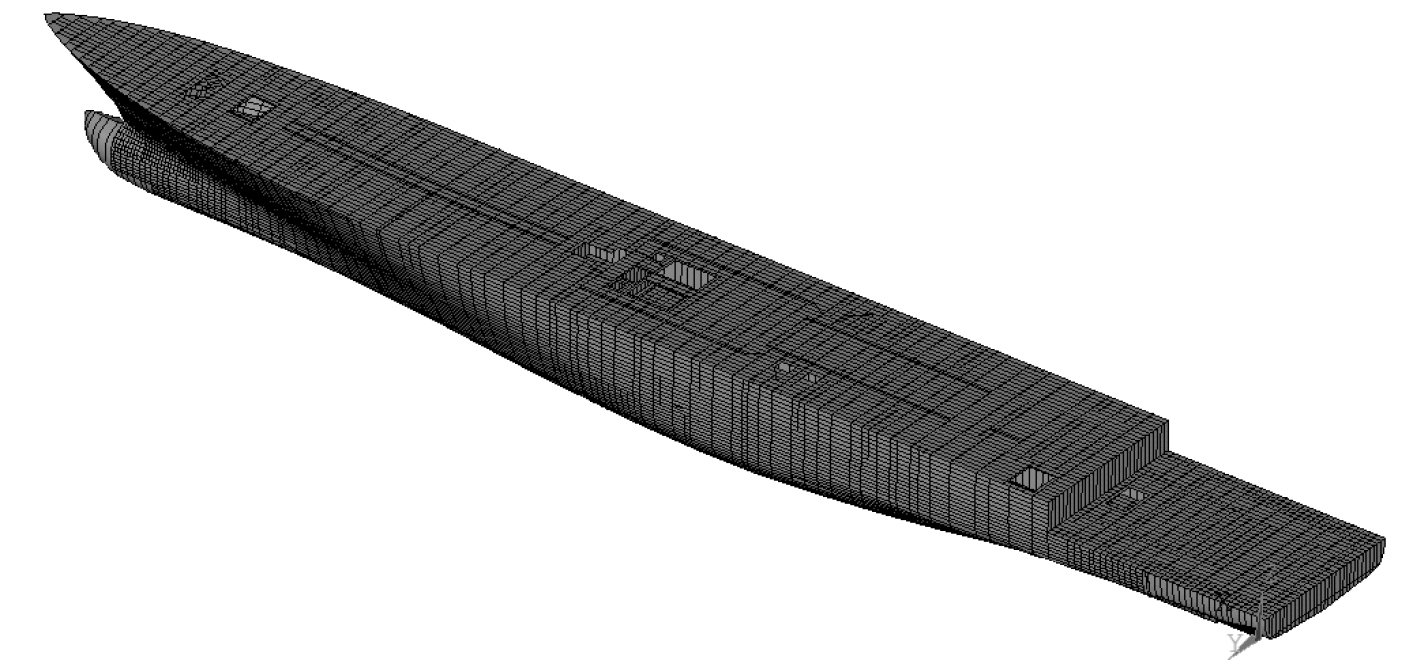图 4 船舶有限元模型 Fig. 4 Finite element model of ship
2.3 计算结果及对比分析表 1 不同计算方法计算全船固有频率结果对比 Tab.1 Comparison of results of natural frequency of whole ship calculated by different calculation methods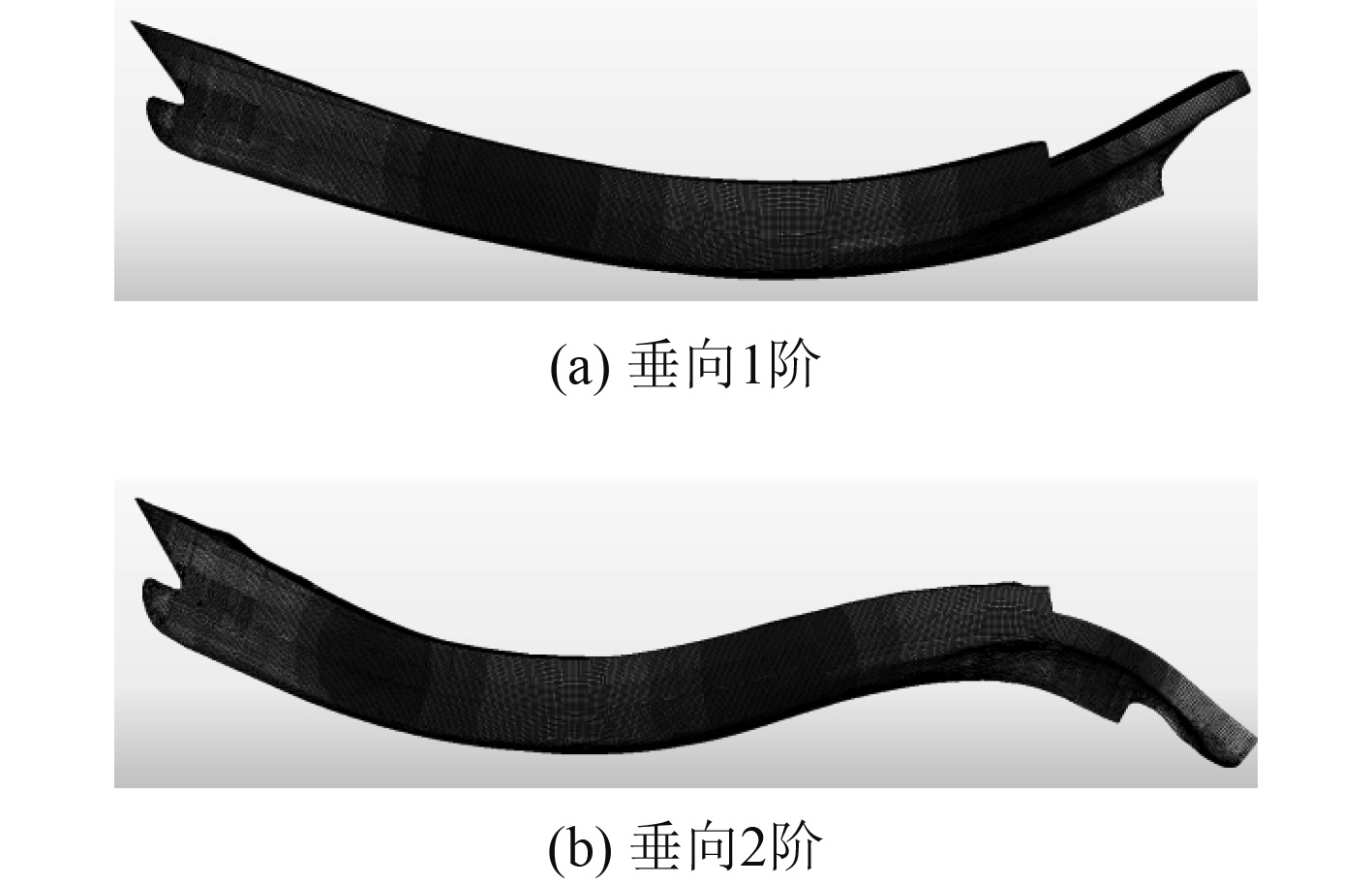图 5 垂向振型图 Fig. 5 Vertical mode diagram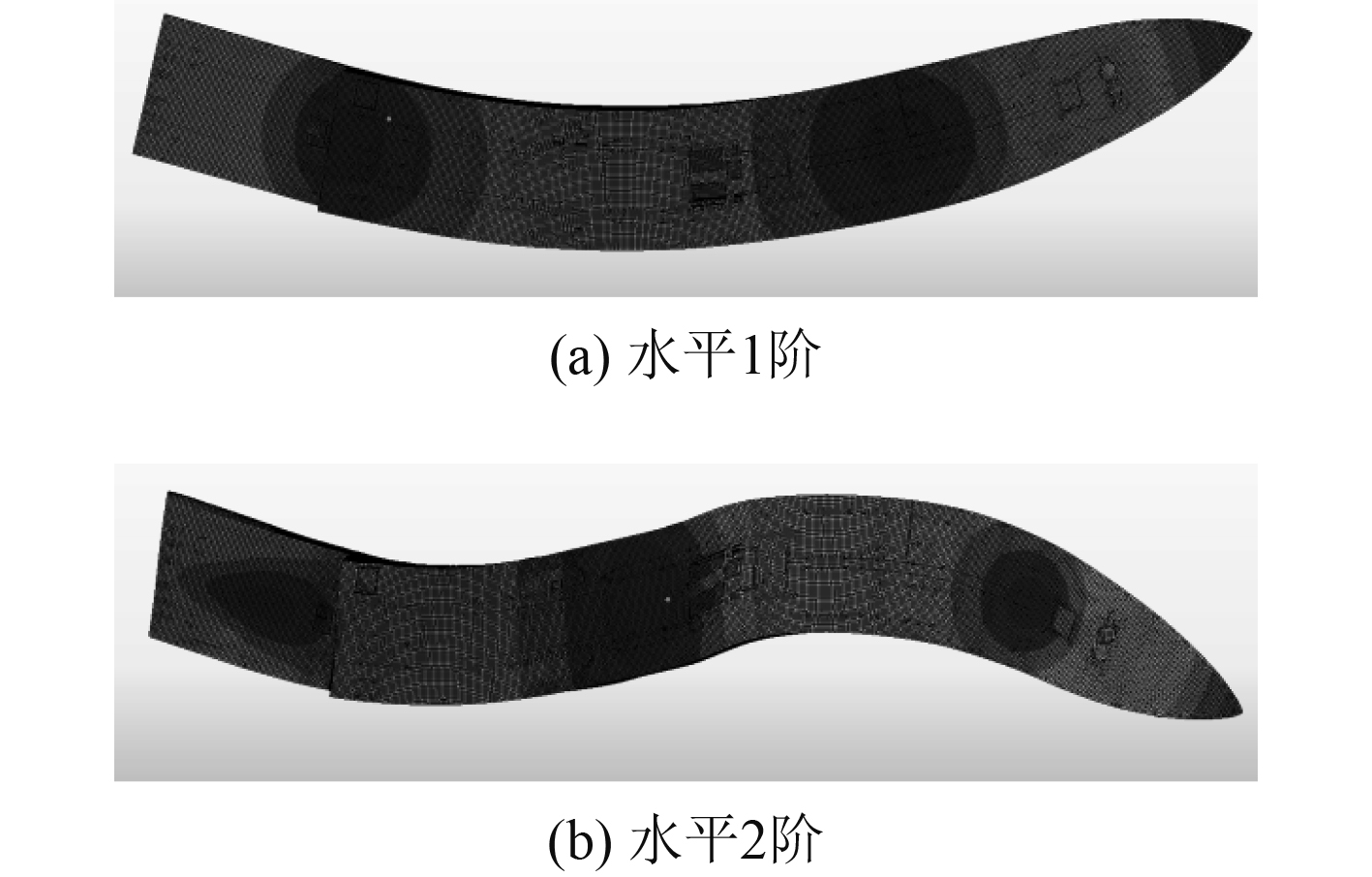图 6 水平振型图 Fig. 6 Horizontal mode diagram
3 重心高度对固有频率的影响表 2 全船不同重心位置的固有频率值 Tab.2 Natural frequency values of different barycenter positions of the whole ship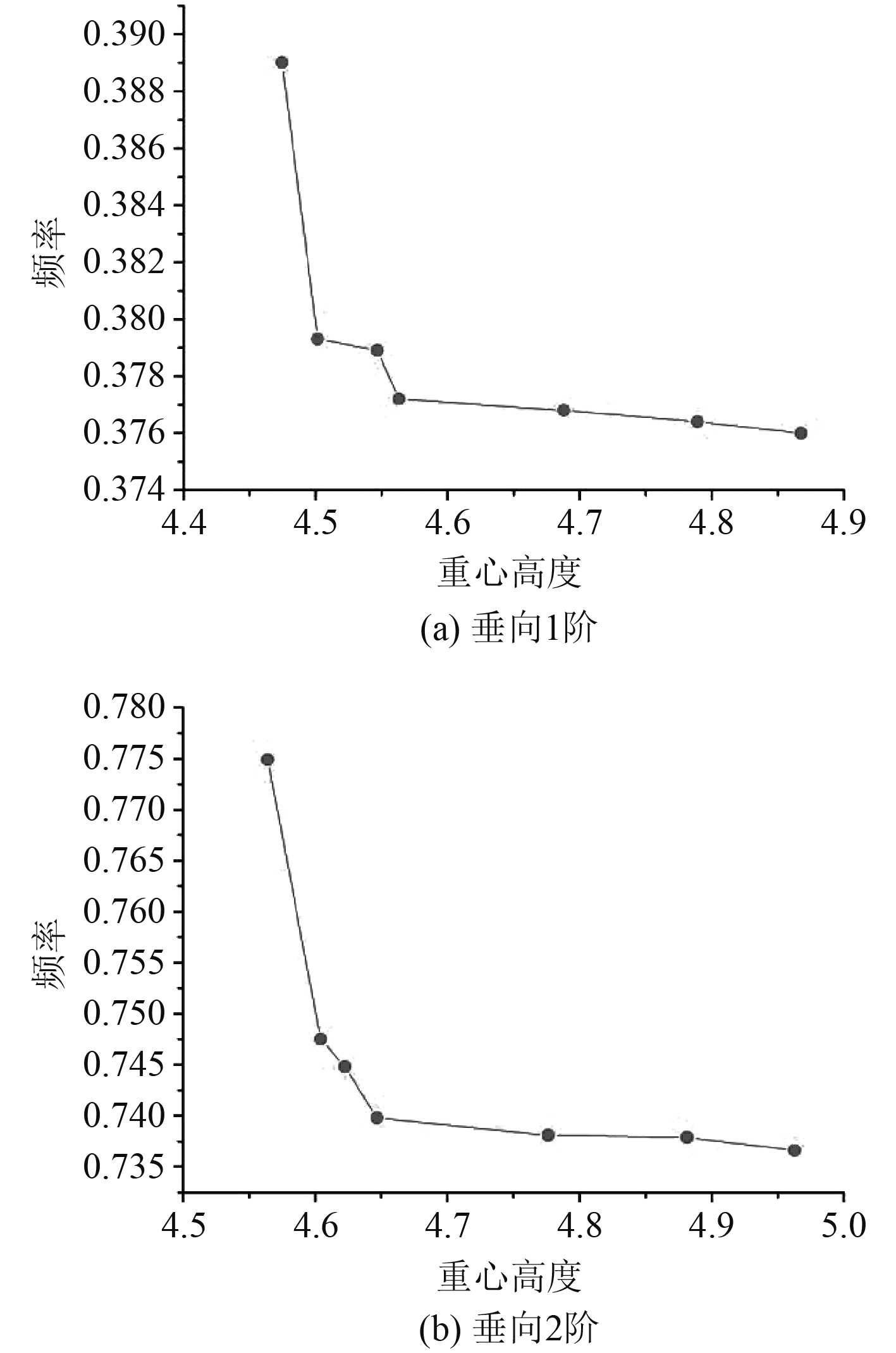图 7 全船垂向固有频率值随重心位置变化曲线 Fig. 7 Vertical natural frequency of the whole ship with the position of the center of gravity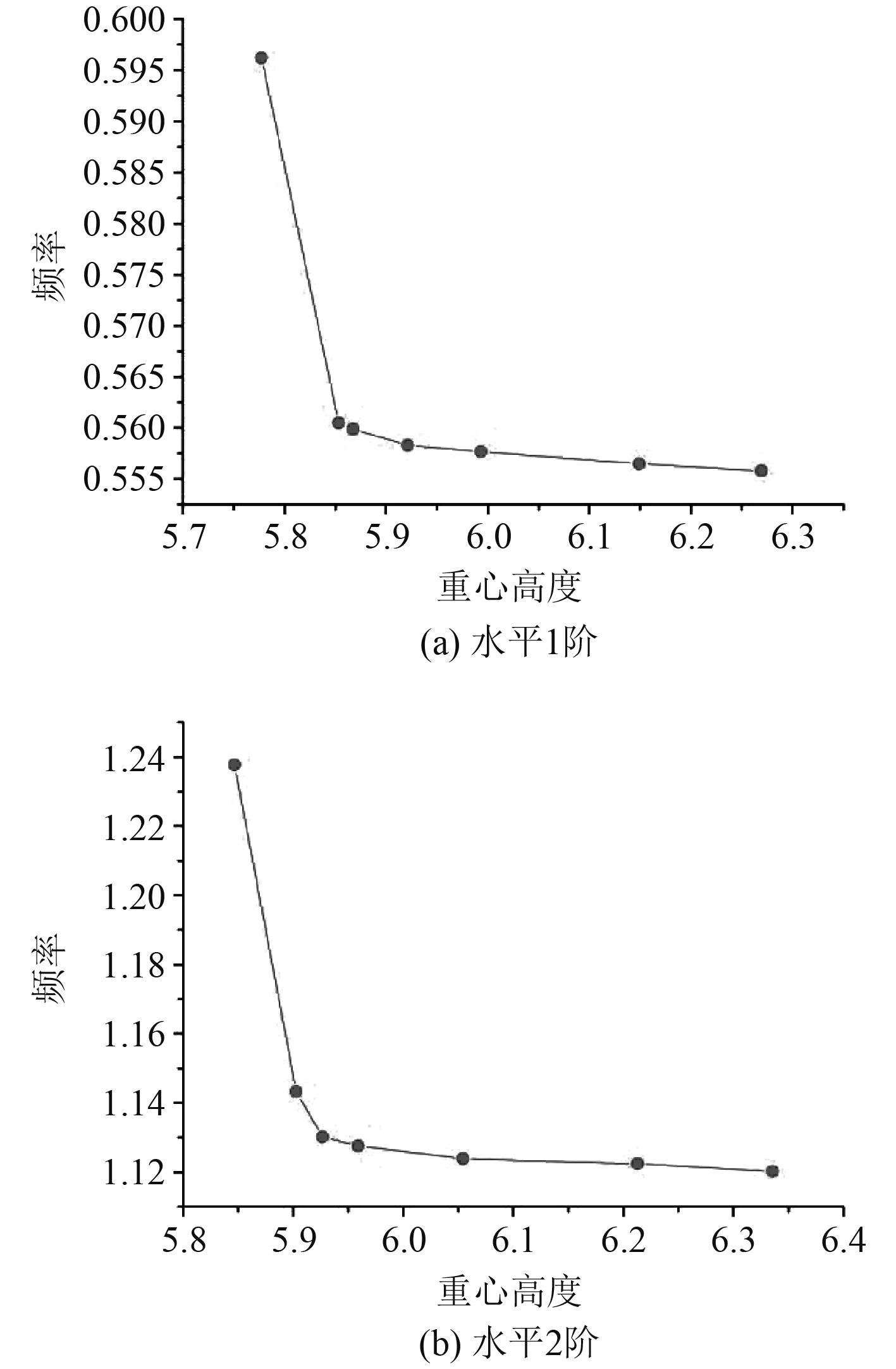图 8 全船水平固有频率值随重心位置变化曲线 Fig. 8 The horizontal natural frequency of the whole ship varies with the position of the center of gravity

4 结　语

1）本文所采用的3种方法均能较为准确计算全船的固有频率，经验公式法较为简便但计算结果仅限前几阶模态且精度不高，一般用于估算结果进行对比验证；附加质量法较为精确，并易于修改，但计算附连水较为繁琐；声固耦合的结果较经验公式相对精确，较附连水的方法更为简单，但受计算水域的大小和水域网格大小较为敏感，需要一定的理论知识和经验才能得到精确的振动频率值。

2）计算全船固有频率时，提高重心高度会降低固有频率的大小，反之减小重心高度则会增大固有频率的大小，且随着重心高度的不断增大，变化趋势会趋于平缓，相对而言，固有频率对较小的重心高度变化更为敏感。

  中国船级社. 船上振动控制指南[S]. 北京: 人民交通出版社, 2000: 174–189, 225–227  王鹏, 李天匀, 朱翔, 等. 浅水域中圆柱壳固有振动特性分析[J]. 中国造船, 2016, 57(3): 72-82. DOI:10.3969/j.issn.1000-4882.2016.03.009  姚熊亮. 船体振动[M]. 哈尔滨: 哈尔滨工程大学出版社, 2004.  姚熊亮, 张阿漫, 许维军. 声固耦合方法在舰船水下爆炸中的应用[J]. 哈尔滨工程大学学报, 2005, 26(6): 707-712. DOI:10.3969/j.issn.1006-7043.2005.06.003  史丰荣. 船体结构总体振动特性预测技术研究[J]. 中国造船, 2013, 54(2): 118-123. DOI:10.3969/j.issn.1000-4882.2013.02.015  金咸定, 夏利娟. 船体振动学[M]. 上海: 上海交通大学出版社, 2010  LEWIS F M. Vibration and engine balance in diesel ship[J]. S. N. A. M. E. , 1927.  LEWIS F M. The intertia of the water surrouding a vibration ship[J]. S. N. A. M. E. , 1929.  何欢. 船舶总体振动的附加水质量算法研究[D]. 大连: 大连理工大学, 2014.  刘强. 小水线面双体船附连水质量及振动特性研究[D]. 大连: 大连理工大学, 2015.  YE R, ZHAO N, YANG D, et al. Bending and free vibration analysis of sandwich plates with functionally graded soft core, using the new refined higher-order analysis model[J]. Journal of Sandwich Structures and Materials, 2020, 23(2): 680−710.